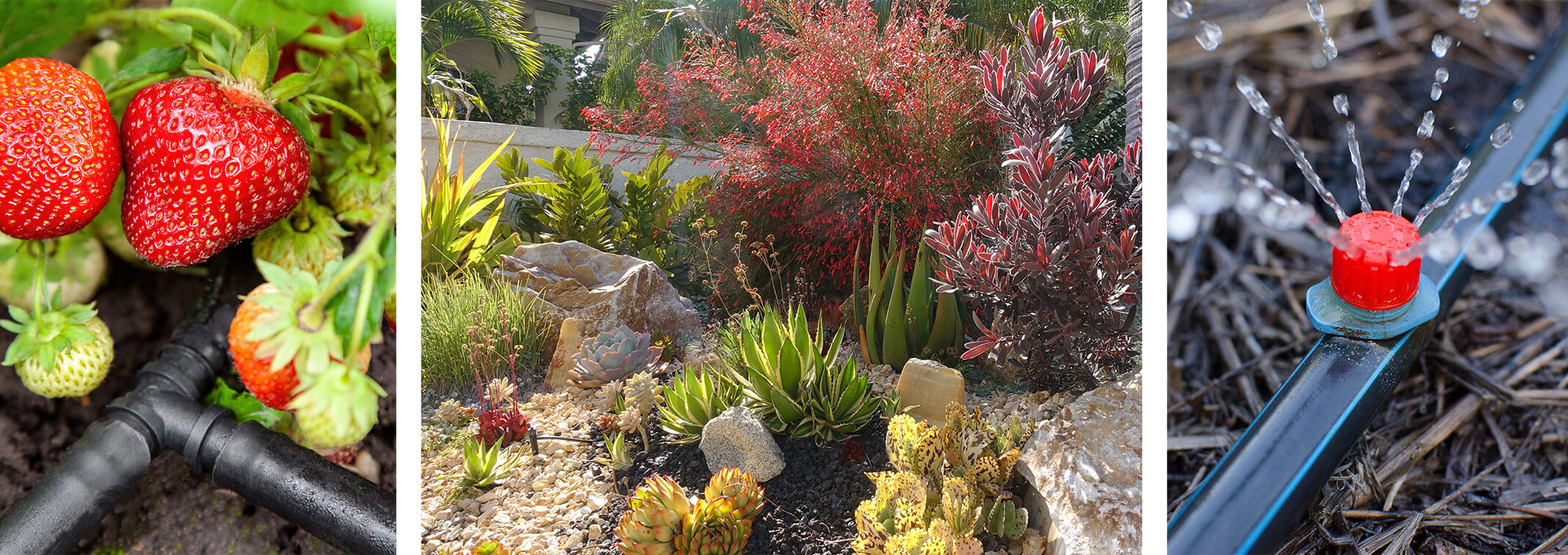# A Seasonal Guide to Drip Irrigation

We all know how important it is to hit the sweet spot with watering in Arizona. Watering just the right amount saves water yet still gives the plants what they need. Drip irrigation is a great way to get accurate watering while reducing the loss through evaporation. So how much water do you use in different seasons?

Here’s a detailed summary of our highly recommended guide by Water Use It Wisely!

## Drip Irrigation Part 1: How Much?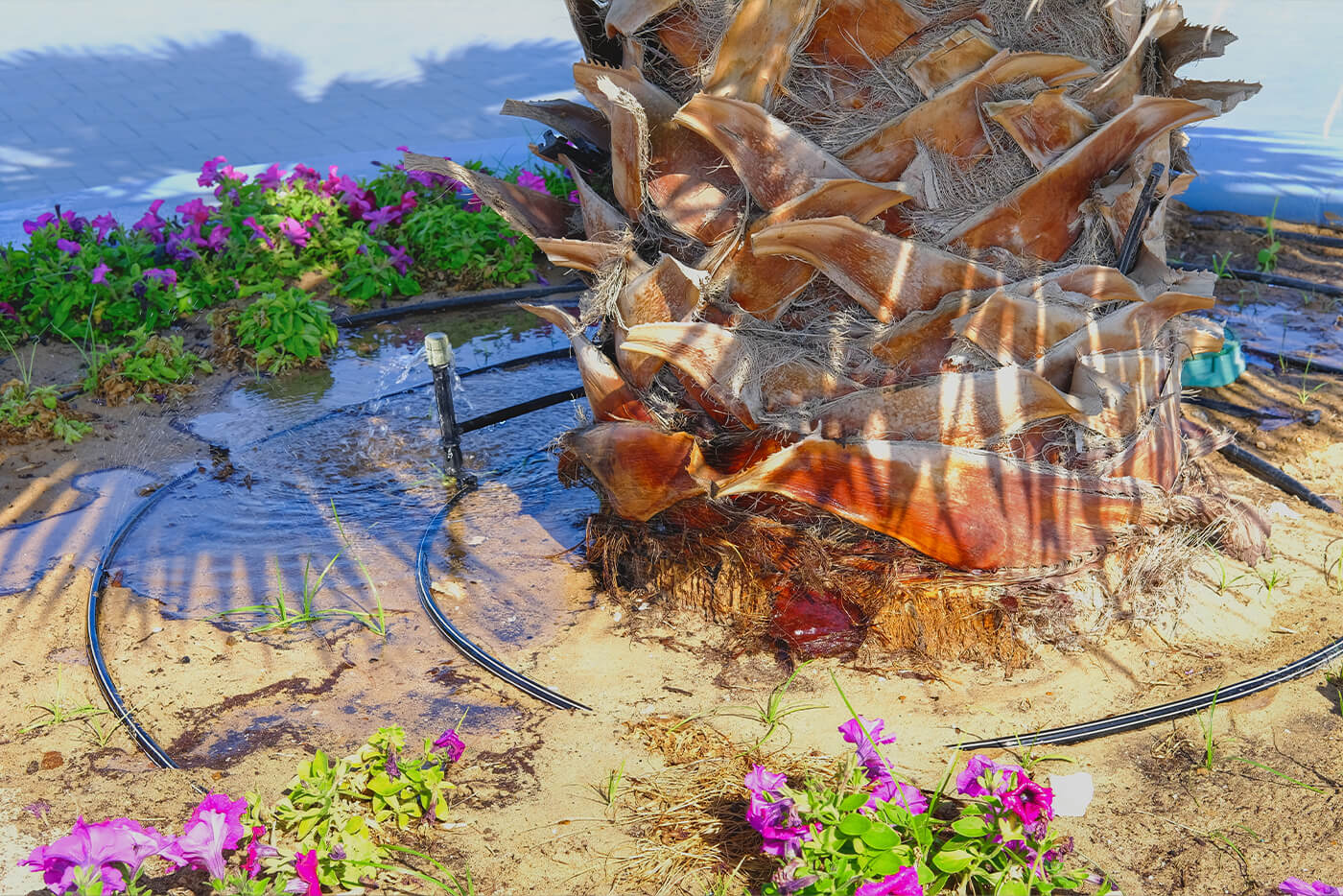Before we look at the seasonal changes, let’s start with understanding how much water your different types of plants need and how we can give it to them with drip irrigation.

### Watering Depth

As a general rule, small plants such as cacti, ground covers, and perennials need their roots to be watered to a depth of 1 foot, while shrubs need 2 feet of watering, and trees need their roots soaked to a depth of 3 feet; this is called the 1-2-3 rule.

Naturally, the next question is: how much water is needed to soak the roots to the appropriate depth? Use a sharpened piece of rebar to probe the soil. Wet soil will allow the rebar to slide into the ground easily. Alternatively, we have a handy chart to tell you how much water you need for each type and size of plant.

GALLONS OF WATER NEEDED TO WET THE ROOT ZONE

 Plant Canopy Diameter (ft) 1’ 2’ 3’ 4’ 5’ 6’ 7’ 8’ 9’ 10’ 11’ 12’ 13’ Trees 1.5 5 11 16 22 26 38 59 85 115 150 190 235 Shrubs 1 4 8 12 17 20 Small Plants 0.5 2 3.5 5 7 9

Source: “Landscape Watering By the Numbers: A Guide for the Arizona Desert,” p.10 Water Use It Wisely.

So, how do you arrange your drip irrigation system to ensure that each plant gets the correct gallons of water? We need to do a bit of simple math to find the answer. Let’s go over the other numbers we need to know.

• Time: We recommend giving a plant its needed water in a 2-6 hour timeframe. You don’t want to stretch it longer than that; otherwise, the water will evaporate, and it will be harder to measure. Keep this in mind as we piece together the rest of the equation.
• Flow Rate: The flow rate of drip irrigation emitters is measured in gallons per hour and usually ranges between 1-4 gallons per hour.
• Number of Emitters: Each emitter has a specific flow rate, but you can increase the total flow rate by adding emitters around the plant you’re watering. Placing two emitters around a plant doubles the number of gallons per hour. Placing three emitters triples the number of gallons per hour, etc.

####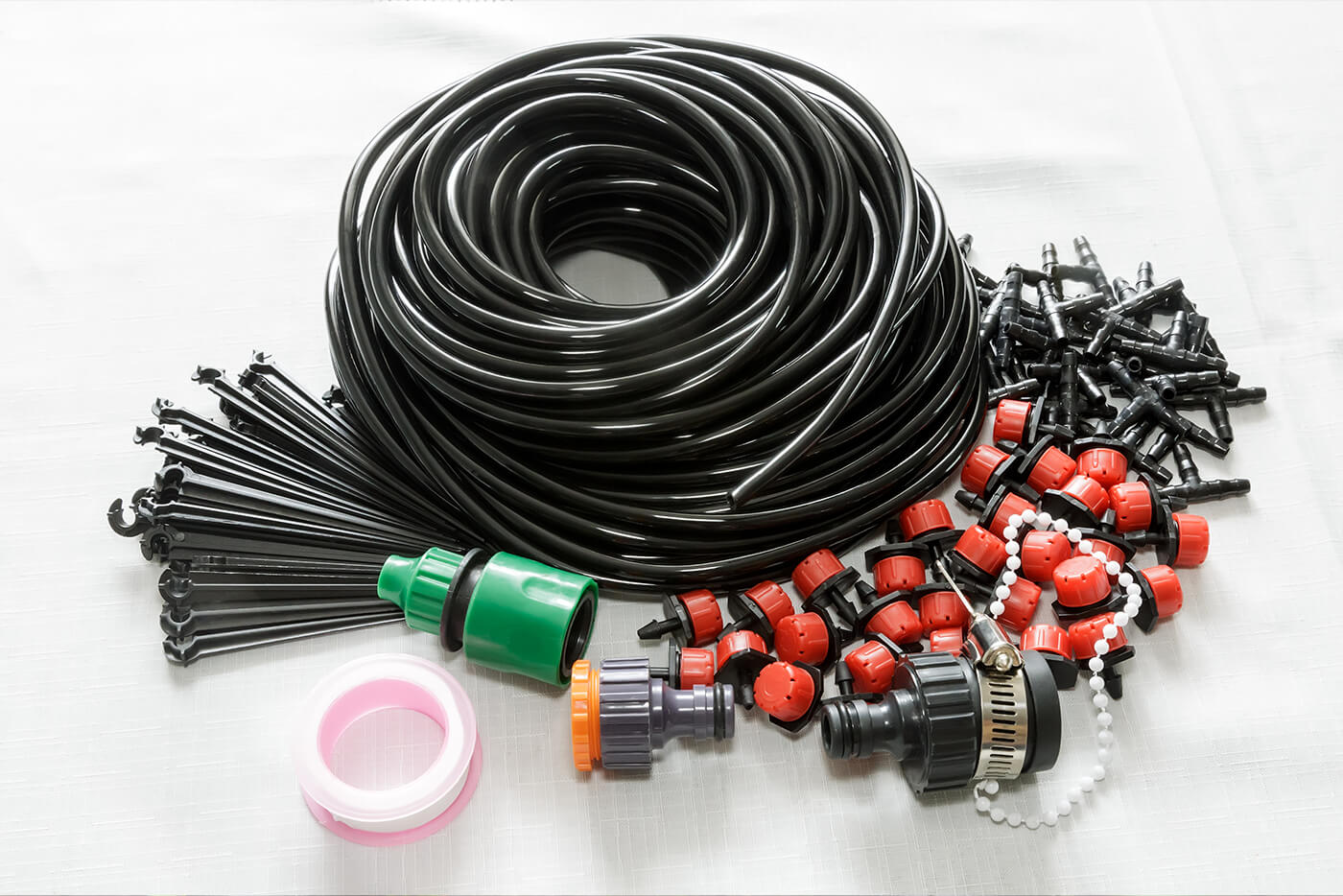How to Long to Water: A Simple Calculation

The big question around using a drip irrigation line is: how long do we need to water each plant? We can easily calculate the answer using the information above and the following equation:

Total Hours = Water Needed / (Number of Emitters X Flow Rate)

Example 1:

Let’s do an example together. Say, for instance, we have a 6-foot wide shrub. From Table A, we see that it needs 20 gallons of water. Now we ask, what’s our flow rate and how many emitters do we have? Let’s say we have two emitters that each have a flow rate of 2 gallons per hour. Since 2 X 2 = 4, we’ll have a total flow rate of 4 gallons per hour.

So how many hours will it take to give it 20 gallons?

Total Hours     = 20 / 4

= 5

Therefore, we’ll need 5 hours of watering at that flow rate and the number of emitters. As a final check, let’s ask: does that fall within our 2-6 hour watering window? Yes, it does.

Example 2:

Let’s do another example. Suppose we have a 10-foot wide tree that needs 59 gallons of water. Let’s say we have four emitters that each have a flow rate of 2 gallons per hour. Let’s put these numbers into the equation and do the math.

Total Hours     = Water Needed / (Number of Emitters X Flow Rate)

= 59 / (4 X 2)

= 59 / (8)

= 7.4

The answer is 7.4 hours, but we have a problem. That exceeds the recommended 2-6 hour timeframe. So, let’s add another emitter to the equation and see where that takes us.

With 5 emitters at a flow rate of 2 gallons per hour, the equation looks like this.

Total Hours     = 59 / (5 X 2)

= 59 / (10)

= 5.9

So with five emitters at this rate, we would have to water for 5.9 hours to reach 59 gallons of water. That fits within the time window!

## Drip Irrigation Part 2: Frequency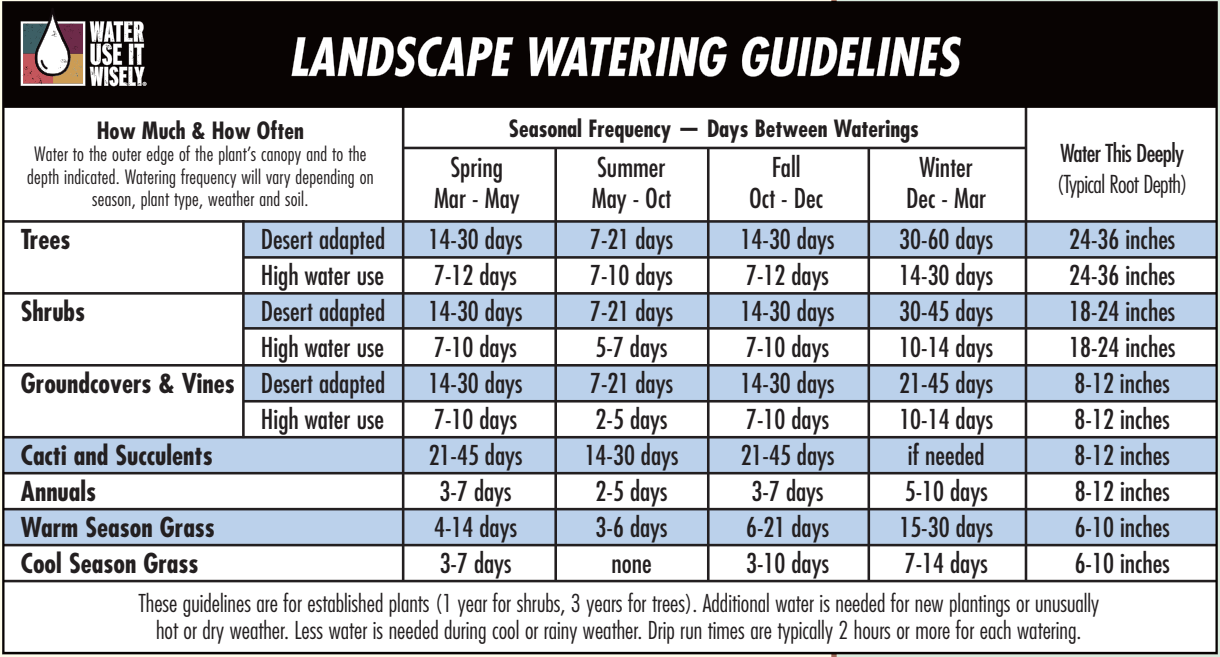The frequency you’ll need to water will change throughout the seasons. Plants need the most frequent watering in the summer and much less frequent in the winter. The frequency also changes depending on the type of plant, whether it’s a desert-adapted plant or high water use plant. Here’s a chart to help you figure out the correct watering frequency in each season.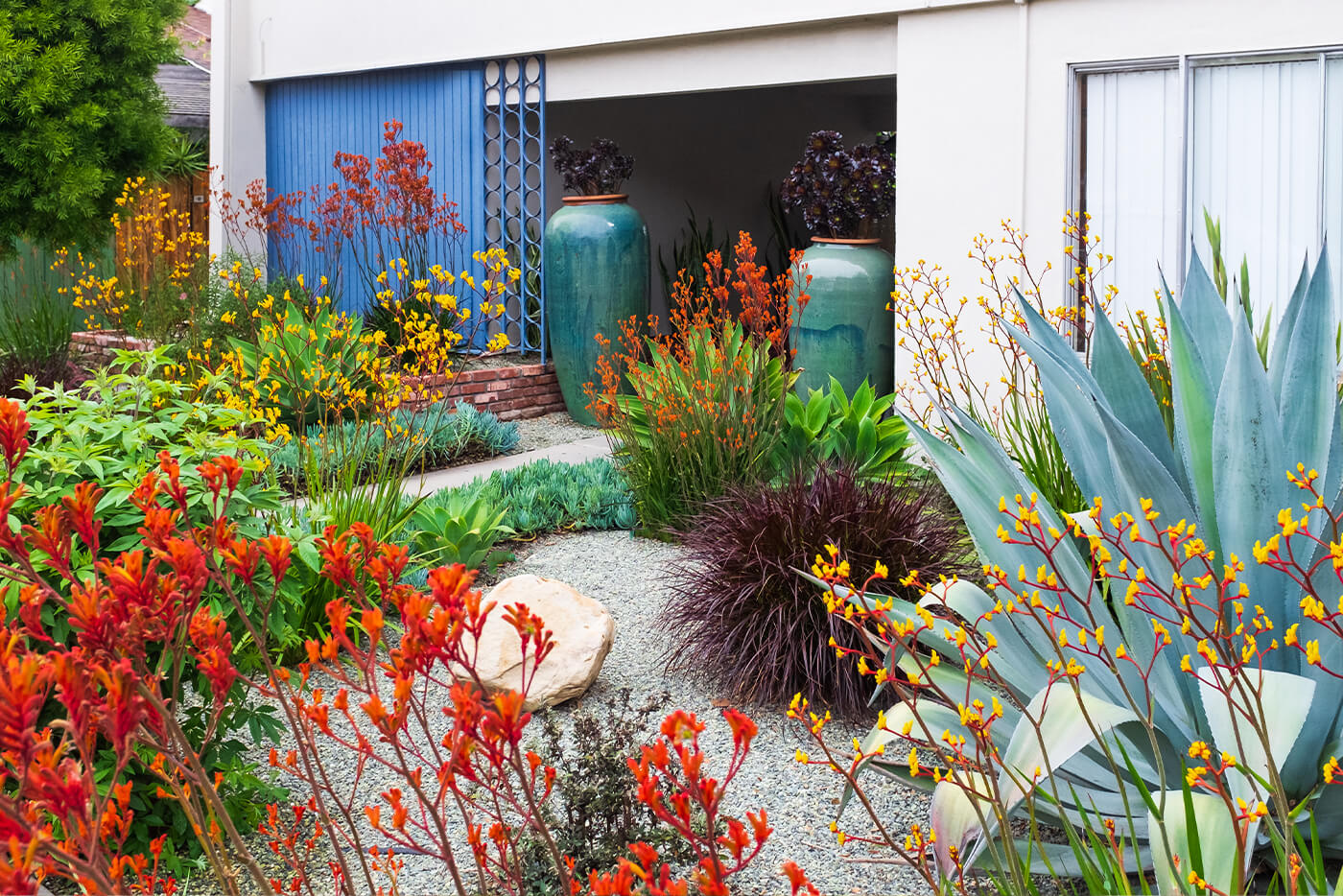Landscape Watering Guidelines chart (image) taken directly from “Landscape Watering By the Numbers: A Guide for the Arizona Desert,” p.13 from Water Use It Wisely.

With this chart, and the simple calculation described above, you can know how much to water your plants and how frequently using an irrigation system. Keep in mind that weather conditions and soil type also impact how much water a plant needs!

If you have more questions or need help setting up your system, feel free to contact our garden centers or check out the source material for this blog at Water Use It Wisely!

At SummerWinds, We Guarantee Success!